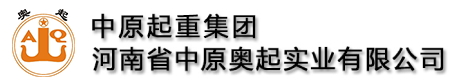全国统一服务热线:13782575673

# 龙门吊大车端梁设计

### 来源：未知   发布时间：2021-03-18 11:01  点击量：

对该台300t的小车采用两组台车8个车轮的结构型式，大车采用8组台车16个车轮的结构型式，在大车轨道面上传递到大车端梁上的受力计算见图4-55。得根据设计得G小=580000N;Q=3000000N;S=33500mm;a=1200mm;b1=2300mm根据用户给定参数(图4-56)，得P大1、P大2。小车端梁各危险截面的受力，见图4-57，图4-57中的P1就是图4-56中的P大1;P4就是图4-56中的P大2。

根据大车主梁和端梁设计：L=4000;L0=2090mm，C=563mm;Gq=1380000N

即PZ=Gq/2=1380000/2=690000NP大1≈P大2，考虑“三合一”减速器的布置，将主动轮布置在大车端梁里侧，P大2/2为大车的最大轮压。根据大车端梁设计：L1=690mm;L2=1488mm;L=4000mm;h1=646mm;h2=1040mm;h=1076mm;t1=20mm;t2=24mm;I1x=2.19×109mm4;S1=4974224mm3;I2x=6.59×109mm4;S2=8739188mm3;y21=651mm;I3x=13.13×109mm4;S3=14019728mm3;y31=576mm;I4x=2.19×109mm4;S4=4974224mm3。M2=P1L1=9.79×105×690=6.76×108kN·mm

M3=P4(L-L1-L2)=9.69×105×(4000-690-1488)=17.66×108kN·mm大车端梁截面符合要求。

关键词：龙门吊